# 从概率分布函数中抽取随机数

2016年 9月 15日

### 概率分布函数简介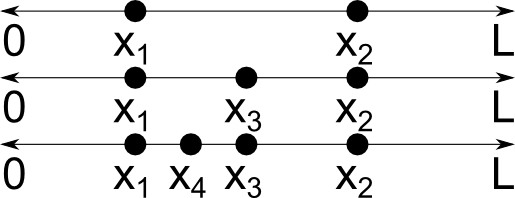(1)

f(x) = \left\{
\begin{array}{cc}
0 & x\leq 0\\
1 & 0 \textless x \textless 1\\
0 & 1\leq x
\end{array}
\right.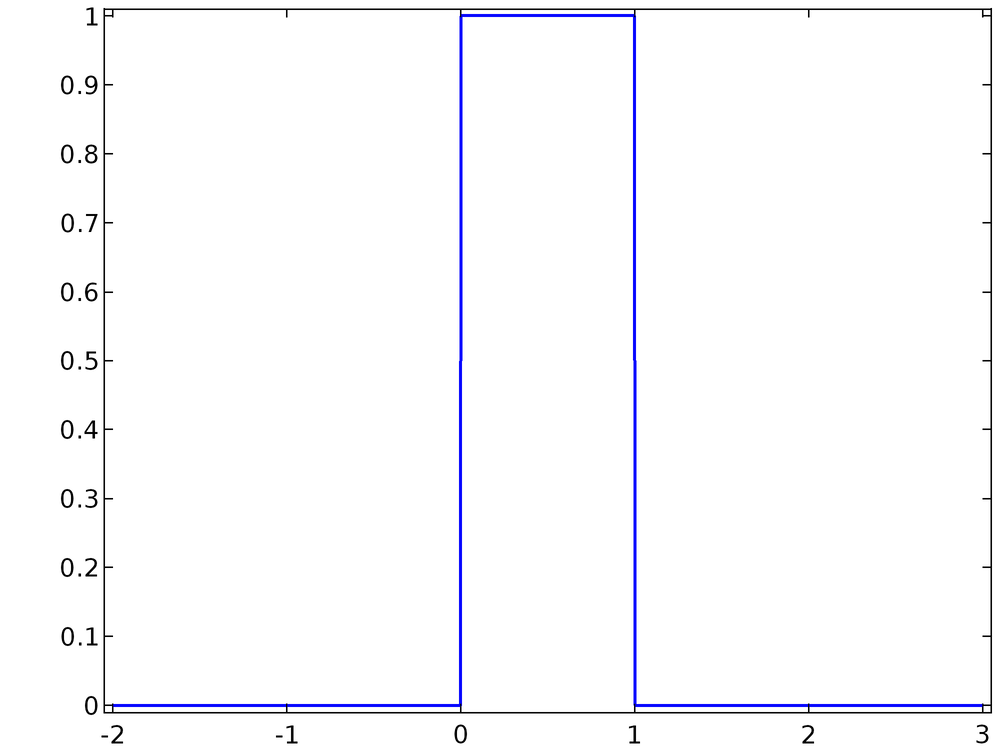\int_{-\infty}^{\infty} f(x)dx = 1

F(x) = \int_{-\infty}^{x} f(x^\prime) dx^\prime

\textrm{lim}_{x \rightarrow \infty} F(x) = 1### 从一维分布中抽样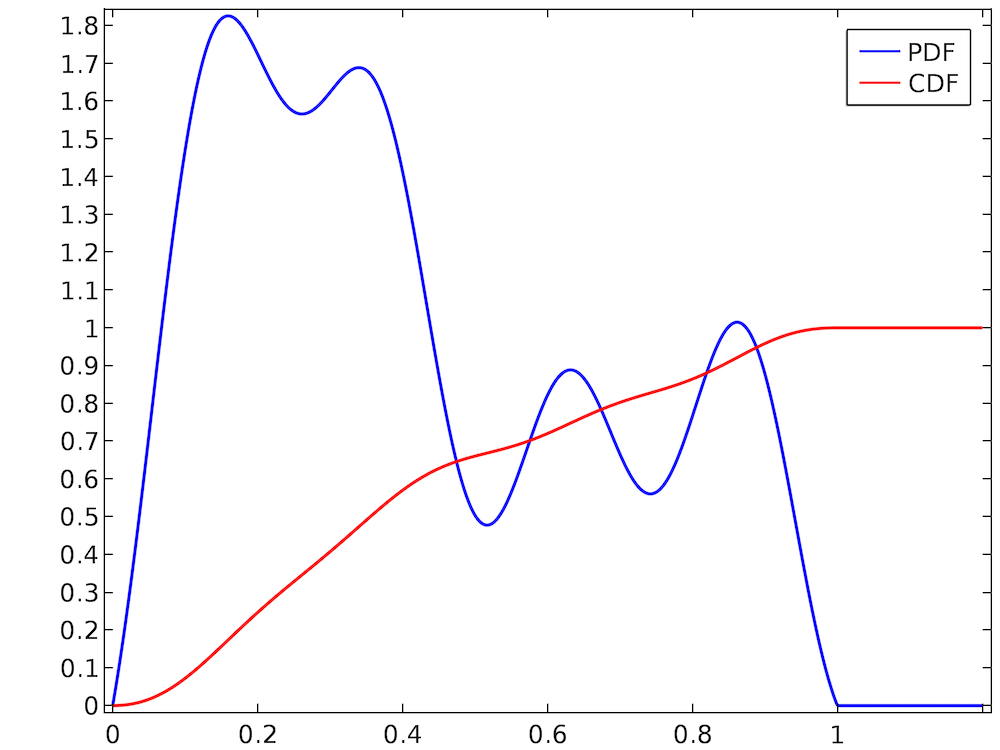1. 若函数 f(x) 尚未被归一化，则对其进行归一化。
2. 求解归一化的 PDF f(x) 的积分，并由此计算出 CDF F(x) 的值。
3. 对函数 F(x) 求逆，得出逆累积分布函数 或者分位数函数 F-1(x)。由于我们对 f(x) 进行了归一化，所以亦可称之为逆正态累积分布函数，或将其简单表述为逆正态 CDF。
4. 将均匀分布的随机数 U 的值代入逆正态 CDF。

### 示例 1：Rayleigh 分布

Rayleigh 分布 经常出现在稀薄气体动力学和束流物理的方程中，其表达式为

(2)

f(x) = \left\{
\begin{array}{cc}
0 & x \textless 0 \\
\frac{x}{\sigma^2} \exp\left(-\frac{x^2}{2\sigma^2}\right) & x\geq 0
\end{array}
\right.

\begin{aligned}
\int_{0}^{\infty} \frac{x}{\sigma^2} \exp\left(-\frac{x^2}{2\sigma^2}\right)dx
&= \lim_{x \rightarrow \infty} \left.-\exp\left(-\frac{x^\prime^2}{2\sigma^2}\right)\right|^x_0\\
&= 1-\lim_{x \rightarrow \infty}\exp\left(-\frac{x^2}{2\sigma^2}\right)\\
&= 1
\end{aligned}

\begin{aligned}
F(x)
&=\int_{0}^{x} \frac{x^\prime}{\sigma^2} \exp\left(-\frac{x^\prime^2}{2\sigma^2}\right)dx^\prime\\
&= \left.-\exp\left(-\frac{x^\prime^2}{2\sigma^2}\right)\right|^x_0\\
&= 1-\exp\left(-\frac{x^2}{2\sigma^2}\right)
\end{aligned}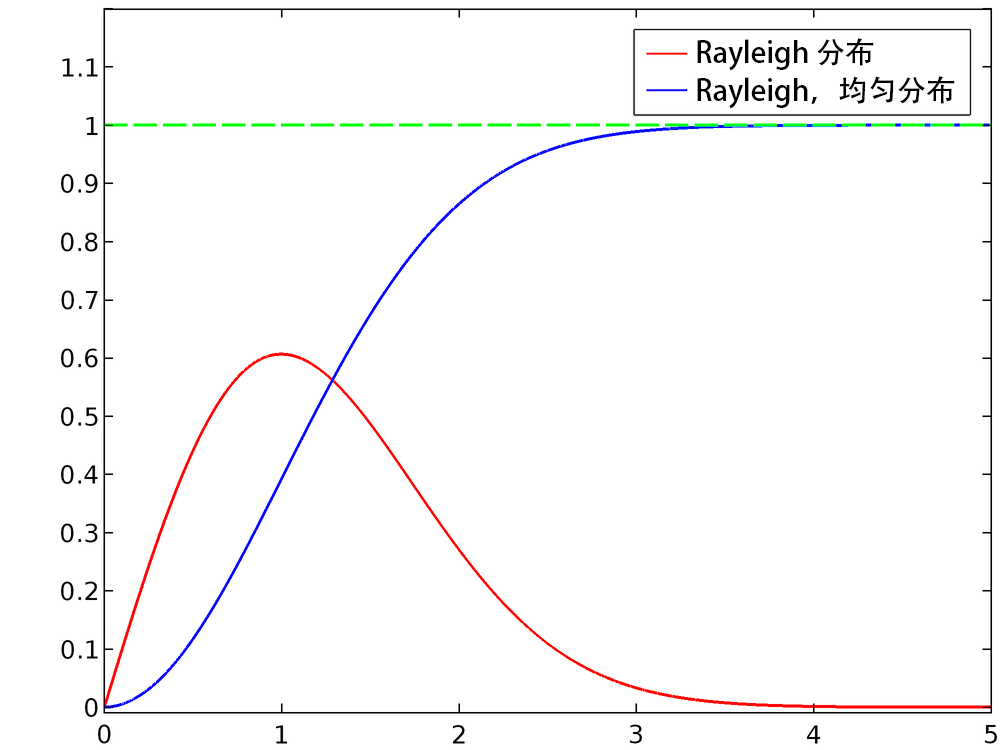\begin{aligned}
y &= F(x)\\
y &= 1-\exp\left(-\frac{x^2}{2\sigma^2}\right)\\
\exp\left(-\frac{x^2}{2\sigma^2}\right) &= 1-y\\
-\frac{x^2}{2\sigma^2} &= \log\left(1-y\right)\\
x &= \sigma \sqrt{-2 \log\left(1-y\right)}
\end{aligned}

x = \sigma \sqrt{-2 \log\left(1-U\right)}

(3)

x = \sigma \sqrt{-2 \log U}

### 在 COMSOL Multiphysics® 中随机抽样

f(x) = \left\{
\begin{array}{cc}
0 & x \leq \mu_u-\frac{\sigma_u}{2}\\
\frac{1}{\sigma_u} & \mu_u-\frac{\sigma_u}{2} \textless x \textless \mu_u + \frac{\sigma_u}{2}\\
0 & \mu_u + \frac{\sigma_u}{2} \leq x\\
\end{array}
\right.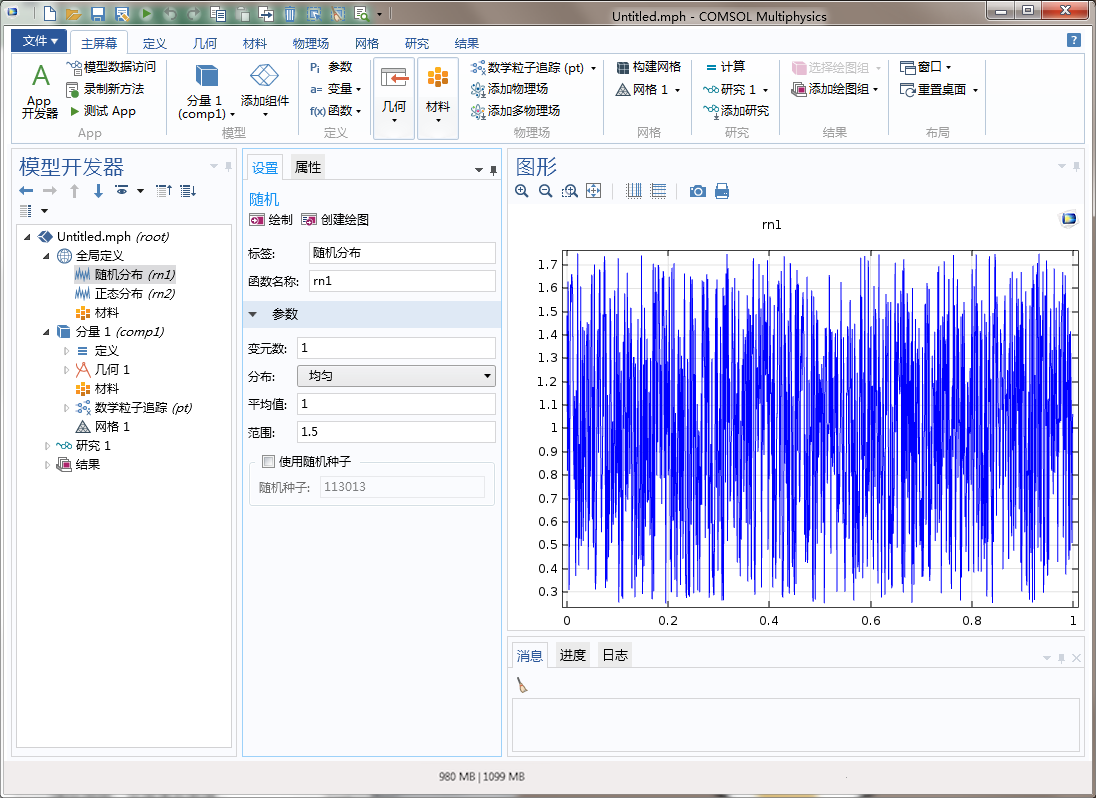f(x) = \frac{1}{\sigma_n \sqrt{2\pi}} \exp\left(-\frac{\left(x-\mu_n \right)^2}{2\sigma_n^2}\right)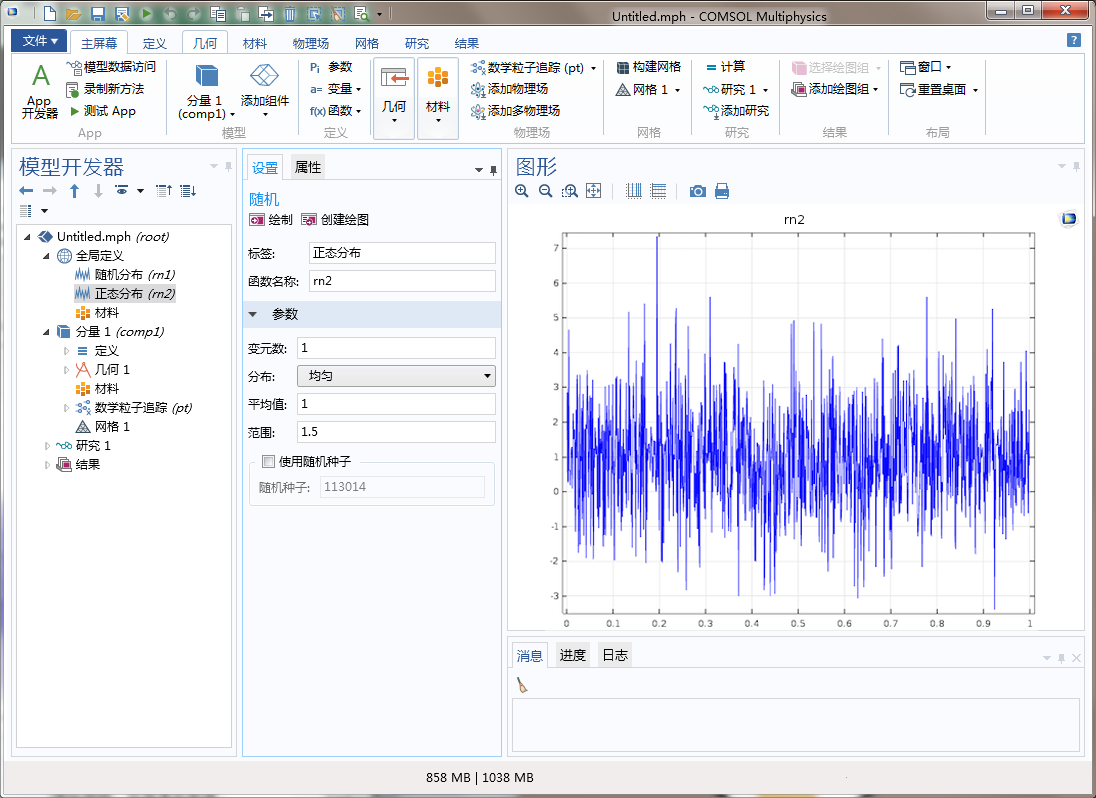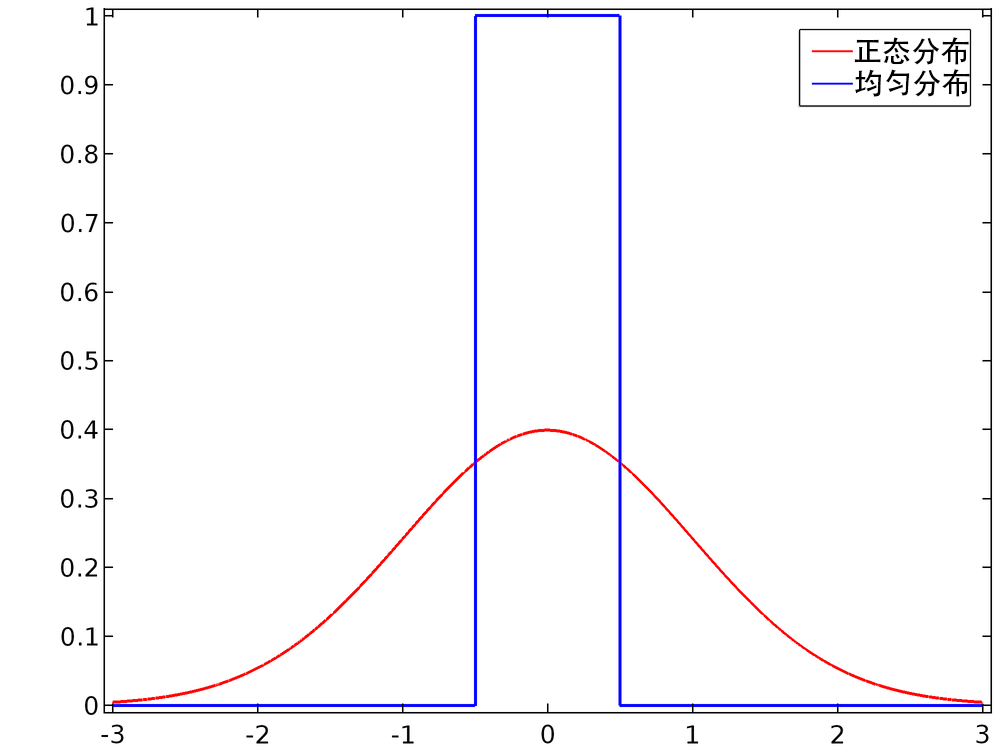1. 使用“随机”函数特征，并将平均值设为 0.5，范围设为 1。
2. 使用内置的 random 函数，并将平均值和范围增加 0.5。

### 随机数，伪随机数与种伪随机种子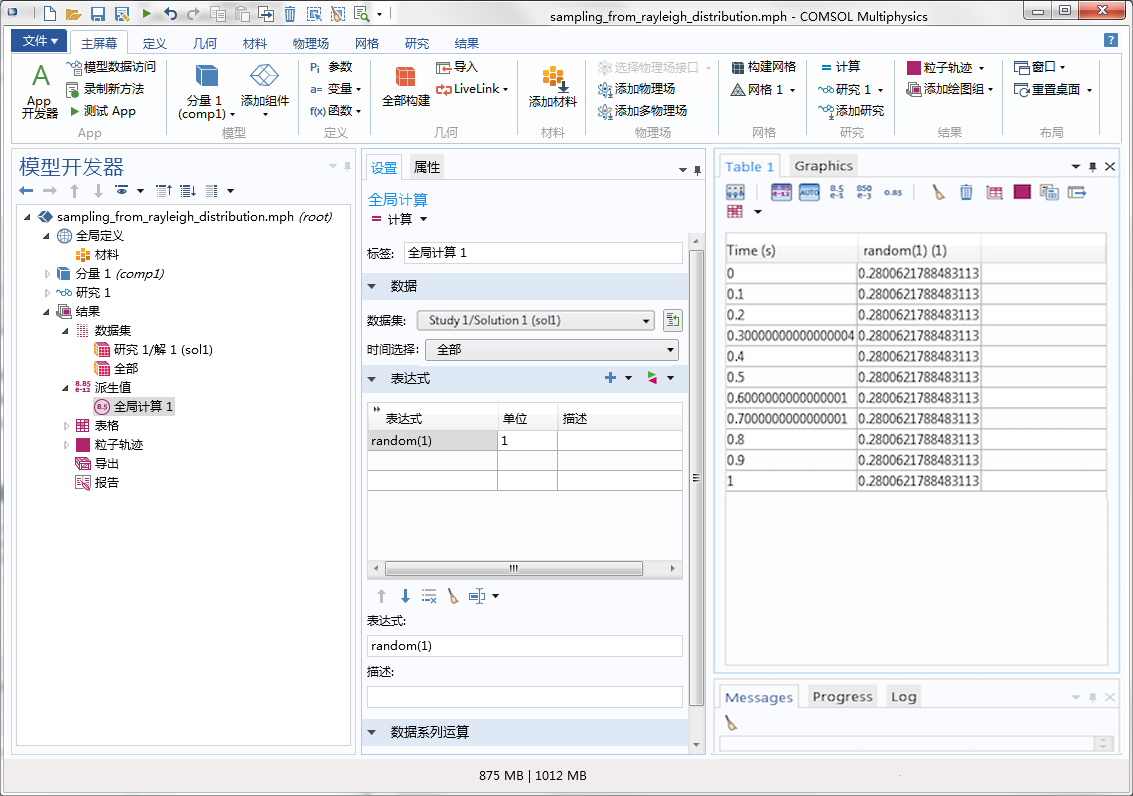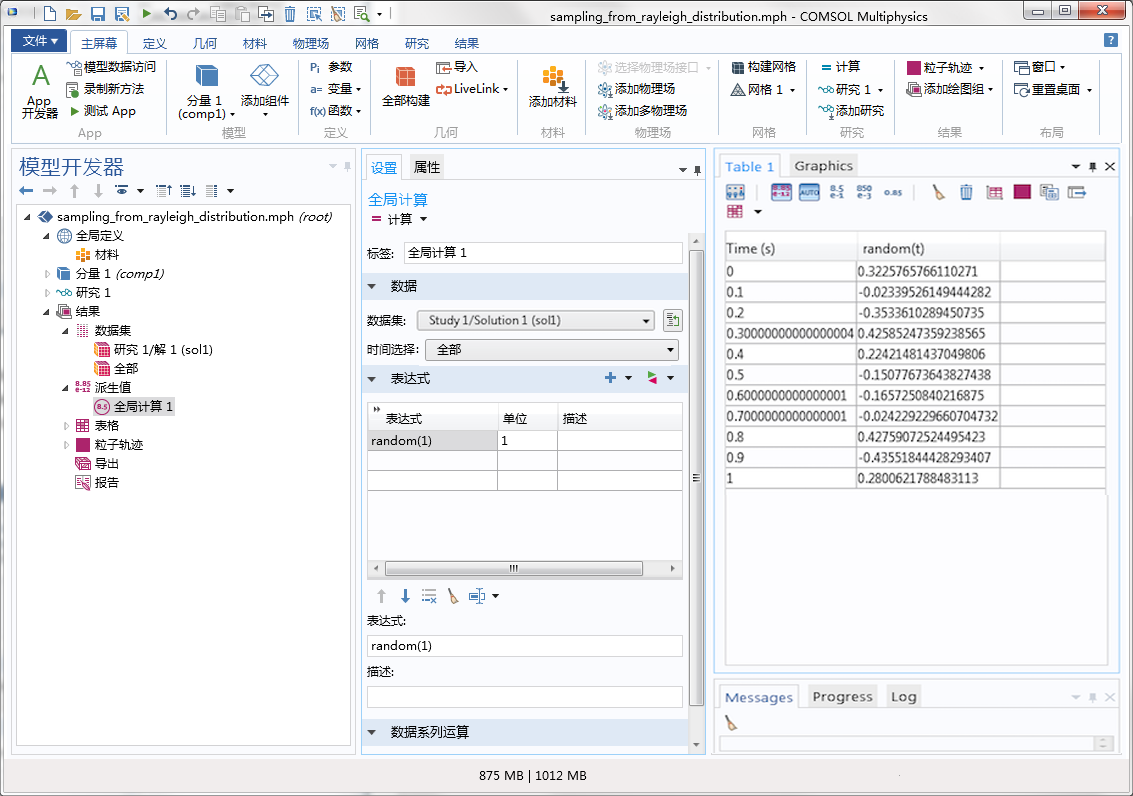### Rayleigh 分布的结果

rn 0.5+random(pt.pidx) Random argument
sigma 3 Scale parameter
val sigma*sqrt(-2*log(rn)) Value sampled from Rayleigh distribution### 插值函数的注意事项

x f(x)
0 0
0.2 0.6
0.4 0.7
0.6 1.2
0.8 1.2
1 0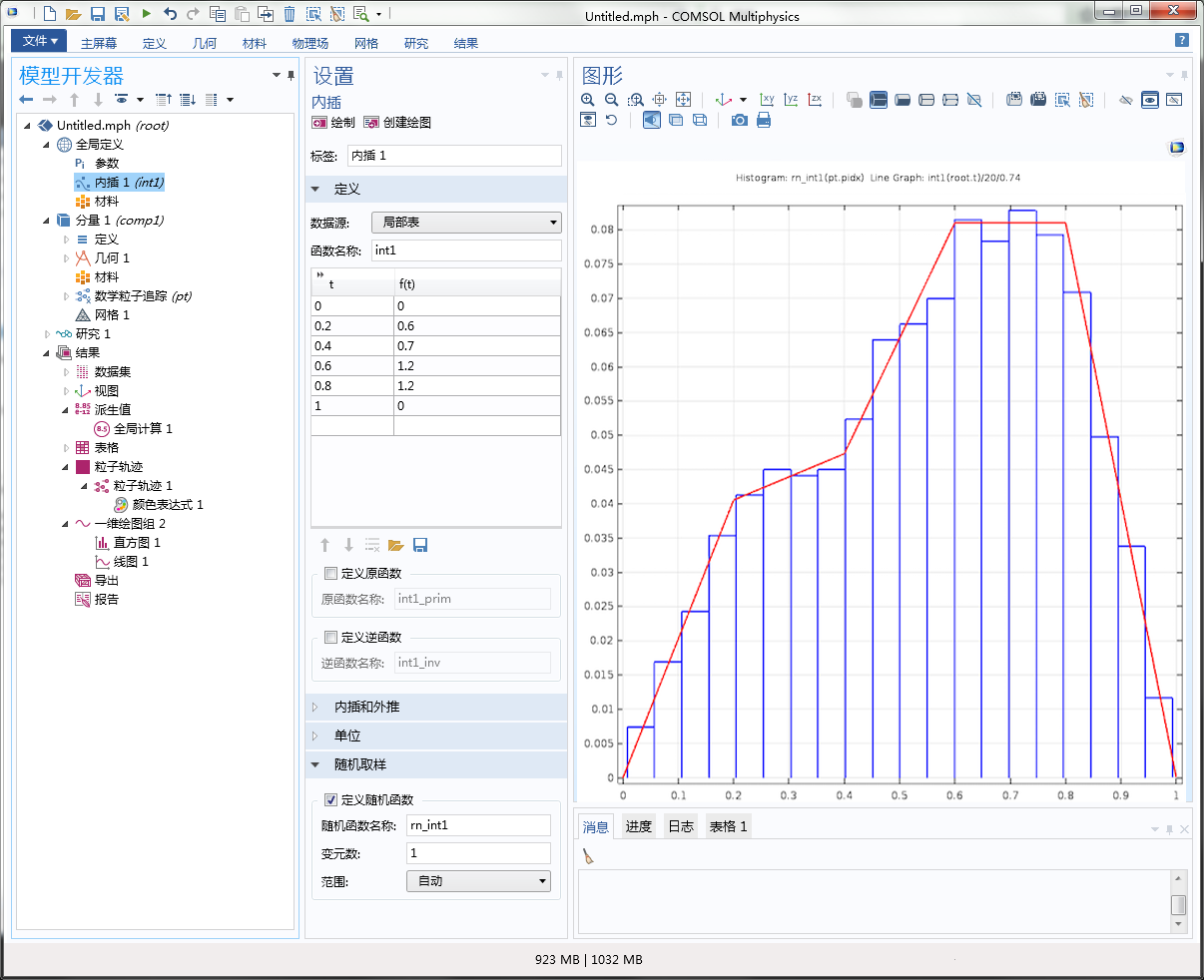### 参考文献

1. Humphries, Stanley. Charged particle beams. Courier Corporation, 2013.
2. Davidson, Ronald C., and Hong Qin. Physics of intense charged particle beams in high energy accelerators. Imperial college press, 2001.

#### 评论 (2)

##### 留言##### Lydia Wong
2018-09-26

Hello, may I want ask for help with random function? I am drawing some circles, maybe 50, in a rectangle with specific length and width. I want to put the circles in random locations in the rectangle. So I plan to put 50 random points first. I can use the random funtion in Global Parameter but it only gets one location. Do you know how to do this?##### 王 刚
2018-10-19

Hi, perhaps you need to read another blog, http://www.comsol.com/blogs/how-to-create-a-randomized-geometry-using-model-methods/